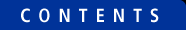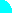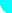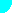# Stream a file into std::vector<char>

###### by Curtis Krauskopf

This is an example program that reads itself using the STL std::copy algorithm. The file is read into a std::vector of char. This example uses istream_iterator, back_inserter and the noskipws manipulator to read the file.

After the file has been imported into a std::vector, information about the vector (its current size, its capacity and its maximum allowed size) are written to the screen.

Next, the vector is changed by prepending a C++ comment line to the std::vector of char.

Finally, the entire contents of the vector is written to the screen using std::copy and an ostream_iterator.

 ```// Stream_Copy.cpp // // Copyright (c) 2009 by Curtis Krauskopf // >>>http://www.decompile.com // // Created: July 15, 2009 by Curtis Krauskopf (cdk) #include #include #include #include #include int main(int, char *) { typedef std::istream_iterator istream_iterator; typedef std::ostream_iterator ostream_iterator; std::ifstream file("Stream_Copy.cpp"); std::vector buffer; file >> std::noskipws; std::copy(istream_iterator(file), istream_iterator(), std::back_inserter(buffer)); // Now that the file has been loaded into our buffer, let's get some // information about the buffer: std::cout << "buffer size(): " << buffer.size() << std::endl; std::cout << "buffer capacity(): " << buffer.capacity() << std::endl; std::cout << "buffer max_size(): " << buffer.max_size() << std::endl; // Insert a C++ comment at the top of the buffer. (just to show we can) std::string helloWorld = "// Hello World\n"; buffer.insert( buffer.begin(), helloWorld.begin(), helloWorld.end() ); // And echo the buffer to std::out (just to show we can) std::copy(buffer.begin(), buffer.end(), ostream_iterator(std::cout, "")); return 0; }``` Figure 1: Stream_Copy.cpp is an example C++ application that reads itself into a std::vector (a vector of type char). The vector is eventually output to the screen using std::cout and an ostream_iterator.

.The output of the program looks like this:```buffer size(): 1313 buffer capacity(): 2048 buffer max_size(): 4294967295 // Hello World // Stream_Copy.cpp // // Copyright (c) 2009 by Curtis Krauskopf // >>http://www.decompile.com // // Created: July 15, 2009 by Curtis Krauskopf (cdk) #include #include #include #include #include int main(int, char *) { typedef std::istream_iterator istream_iterator; typedef std::ostream_iterator ostream_iterator; std::ifstream file("Stream_Copy.cpp"); std::vector buffer; file >> std::noskipws; std::copy(istream_iterator(file), istream_iterator(), std::back_inserter(buffe r)); // Now that the file has been loaded into our buffer, let's get some // information about the buffer: std::cout << "buffer size(): " << buffer.size() << std::endl; std::cout << "buffer capacity(): " << buffer.capacity() << std::endl; std::cout << "buffer max_size(): " << buffer.max_size() << std::endl; // Insert a C++ comment at the top of the buffer. (just to show we can) std::string helloWorld = "// Hello World\n"; buffer.insert( buffer.begin(), helloWorld.begin(), helloWorld.end() ); // And echo the buffer to std::out (just to show we can) std::copy(buffer.begin(), buffer.end(), ostream_iterator(std::cout, "")); return 0; } ```Figure 2: The output of running Stream_Copy.cpp

The first three lines of the program's output show some statistics about the vector. The total size of the file is 1313 bytes and that's exactly how many bytes were used in the vector.

The vector's capacity() is the number of characters that the vector can contain before its size is internally incremented. If you compile and execute this program, the capacity on your application might be different because compiler vendors are allowed increase the space reserved for a vector in any way they want.

The max_size() is the maximum number of characters the vector could ever contain. Your program might show a different value on this line, too.

The program prepended a Hello World comment to the source code just to show that it can.

The vector was written to the screen using an ostream_iterator and std::copy. The constructor for the ostream_iterator used std::cout and an empty string as parameters. This was actually overkill because the std::ostream_iterator<char> template implementation would have defaulted the second parameter to an empty string even if it wasn't provided. In other words, the program would have generated almost the same output if this line had been used:

 ``` // And echo the buffer to std::out (just to show we can) std::copy(buffer.begin(), buffer.end(), ostream_iterator(std::cout));``` Figure 3: This code sample shows an equally valid way to copy the vector to std::cout.

### Conclusion:

Reading a file into a std::vector<char> is very easy. Every byte of the text file is present in the program, including the newline characters. In addition, the std::vector<char> template provides features that a regular character buffer easily provide, such as being able to insert text anywhere in the buffer -- even at the very beginning.

Copyright 2003-2010 The Database Managers, Inc.

Popular C++ topics at The Database Managers:Services | Programming | Contact Us | Recent Updates Send feedback to: